Reduce the fractions to their simplest form, reduce the resulting fractions to their common denominator and then add the numerators together. If the numbers are given as whole numbers plus a fraction, add the whole numbers and fractions each separately and then reduce the result.

Examples

(1) Find the sum of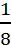,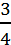of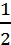and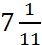The sum is expressed as: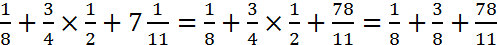.

Following all the rules strictly, Macdonald takes the long route to find the common denominator with the calculation: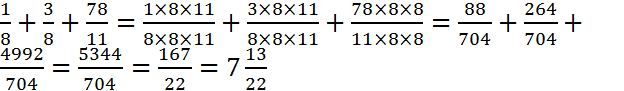The easier route is to add terms with common denominators first and then simply: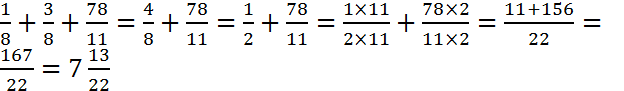(2) Find the sum of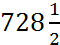,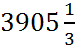and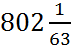.

Add the whole numbers: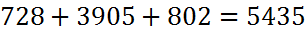.

Add the fractions: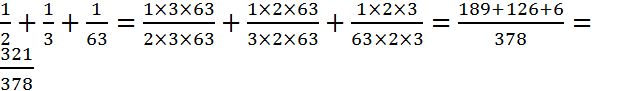.

The sum is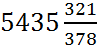.